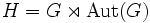# Abelian implies self-centralizing in holomorph

Jump to: navigation, search
This article gives the statement and possibly, proof, of an implication relation between two group properties. That is, it states that every group satisfying the first group property (i.e., abelian group) must also satisfy the second group property (i.e., NSCFN-realizable group)
View all group property implications | View all group property non-implications
Get more facts about abelian group|Get more facts about NSCFN-realizable group

## Statement

Suppose$G$ is an abelian group and$H = G \rtimes \operatorname{Aut}(G)$ is its holomorph. Then,$C_H(G) = G$. In other words,$G$ is a Self-centralizing subgroup (?) of$H$.

Further, since every group is normal fully normalized in its holomorph, this tells us that$G$ is normal, self-centralizing and fully normalized in its holomorph. In short,$G$ is a NSCFN-subgroup (?) of its holomorph, and thus, is a NSCFN-realizable group (?).

## Proof

PLACEHOLDER FOR INFORMATION TO BE FILLED IN: [SHOW MORE]